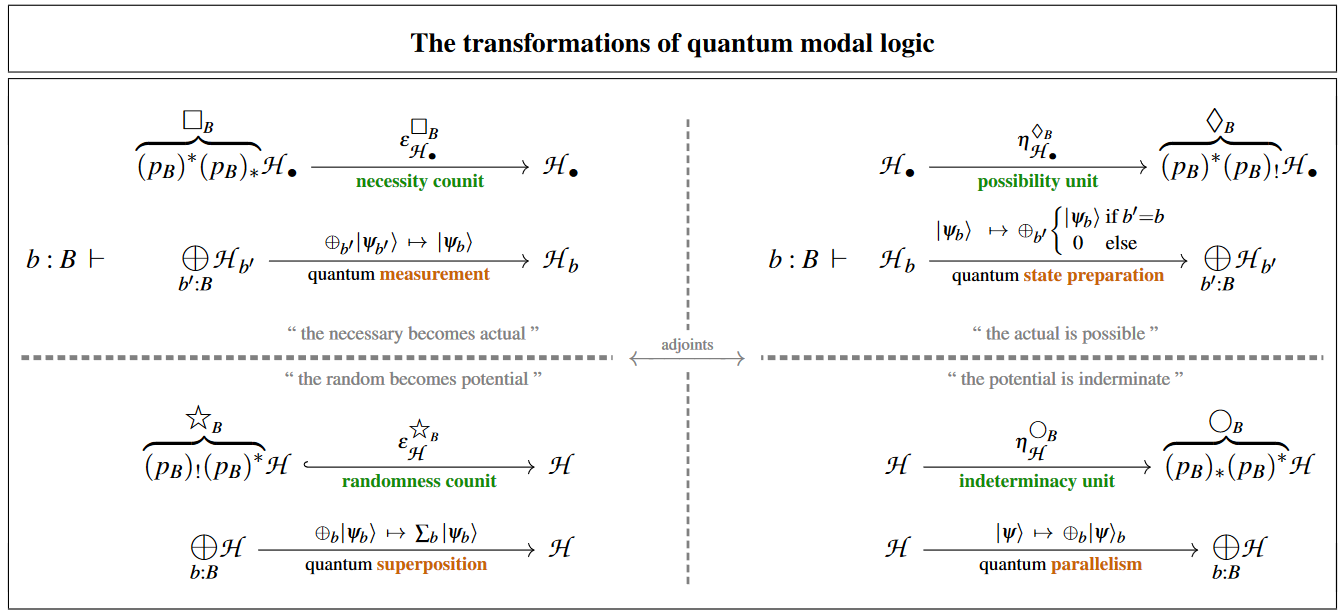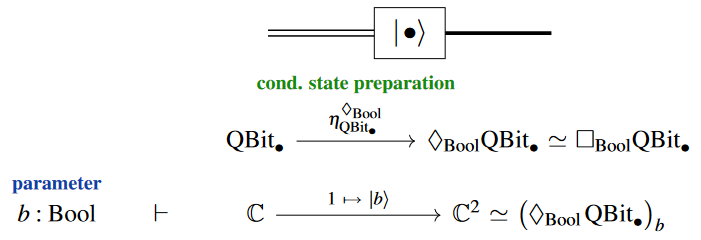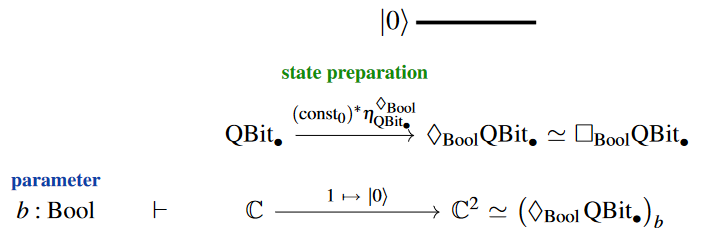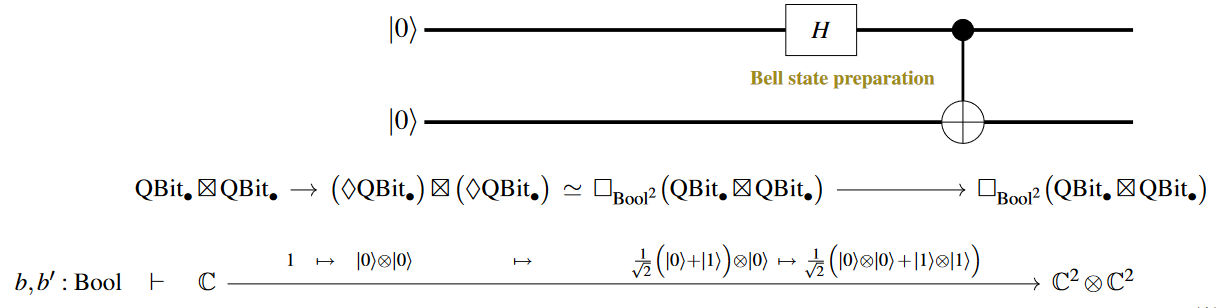Contents

# Contents

## Idea

In quantum physics and especially in quantum information theory, by state preparation one refers to a process (either in actual experiments or theoretically as a kind of quantum gate-operation) which, possibly conditioned on a classical parameter $b$, “produces” a predefined quantum state $\vert b \rangle \,\in\, \mathscr{H}$ in a given space of quantum states $\mathscr{H}$.

For example, for a qbit data type there are, by definition of qbits, two canonical basis states $\vert 0 \rangle, \vert 1 \rangle \,\in\, \mathbb{C}^2$ to be prepared, depending on a classical parameter which may be understood as of boolean type (where we set $Bool \,\coloneqq\, \{0, 1\}$).

The various fundamental quantum physics phenomena at play here may be identified with four modal-units of quantum modal logic (as discussed at quantum circuits via dependent linear types):quantum measurementquantum state preparation
quantum superpositionquantum parallelism

Explicitly, in the language1 of modal quantum logic on dependent linear types (see at quantum circuits via dependent linear types), the conditional preparation of a qbit-state reads as follows:and the unconditional state preparation is of this form:Other states may be prepared by first preparing a qbit-state and then sending it through some quantum gate.

For instance, the Bell state on two qbits may be prepared by first preparing a tensor product of two $\vert 0 \rangle$-states and then sending one through a Hadamard gate and then the resulting two qbits through a CNOT gate:This is used in many common quantum circuits, such as for instance in the quantum teleportation-protocol.

Standard textbook accounts: